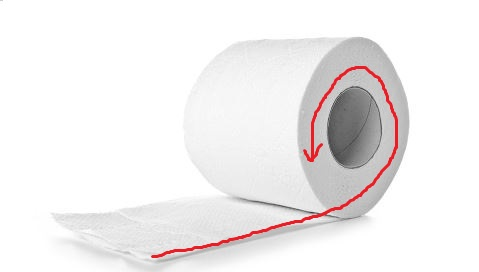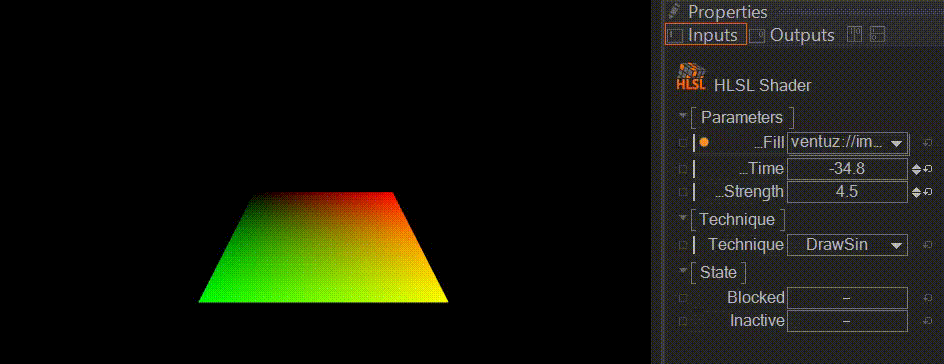# How can I roll up a plane with a vertex shader?

I have plane that I want to roll up using a vertex shader, like this:I found a math demo that shows the kind of curve I want my mesh to follow. I tried implementing this in my shader code, but the results don't form a roll as expected:

Shader " Custom/Rolling "
{
Properties
{
_MainTex ("Base (RGB)", 2D) = "white" {}
_Bend("Bend",Float) = 1
_Strength("Strength",Float) = 1
}

{
Tags {"Queue"="Geometry" "IgnoreProjector"="true" "RenderType"="Opaque"}
Cull Off

Pass
{
CGPROGRAM
#pragma vertex vert
#pragma fragment frag
#include "UnityCG.cginc"

struct v2f
{
half2 texcoord  : TEXCOORD0;
float4 vertex   : SV_POSITION;
};

sampler2D _MainTex;
float _Bend;
float _Strength;

v2f vert(appdata_full v)
{
v2f o;

//v.vertex.y *= ???
v.vertex.y *= exp(_Bend+(v.vertex.x*_Strength)); // something like this but this hasn't twist effect
o.vertex = UnityObjectToClipPos(v.vertex);
o.texcoord = v.texcoord;
return o;
}

float4 frag (v2f i) : COLOR
{

float2 uv = i.texcoord.xy;
float4 tex = tex2D(_MainTex, uv);

return tex;
}
ENDCG
}
}
Fallback Off
}


Instead of a neat roll, the end of my plane shoots up into the sky!• Use the formula from the desmos link to perform the operation from a script (changing the Mesh during Update()). Once it works, figure out if the performance is fine, if it isn't you can move it to the shader. Dec 8 '17 at 10:21
• @Peter thanks but I believe shader is better way , i like to implement rolling by shader , anyway i don't know how can I apply desmos formula. Dec 8 '17 at 10:57
• You won't exactly lose any time by doing it from script first. The big part is creating the mesh and deciding how you want to "bind" the mesh to the transformation, which is easier to code and debug from script, and quite easy to translate afterwards.l To translate the formula, either use one of the plane's coordinates (e.g. x) as p from the desmos formula (which requires you to always calculate the transformed model from the same original model), or set uv3 to p while creating the model (which allows you to work with a single model which you continuously transform). Dec 8 '17 at 11:07
• Nice representation. Dec 8 '17 at 15:57
• Hey did you figure it out ? Apr 2 '20 at 23:53

Here I'm going to assume your geometry is set up like so:

• It's a ribbon of quads extending from z = 0 to z = end

• The z = 0 line will be the outer lip of the roll, and z = end will be the part tucked deepest inside the spiral

• Each quad in the ribbon will span an equal angle when coiled in the roll (but not an equal length of ribbon: the quads in the tightest part of the roll will be smaller) - this gets us a consistent resolution for drawing our curves, and we'll correct for the texture stretching in the shader

• The ribbon is 1 unit wide along the x axis

• The UVs are set up with u/x running across the ribbon, and v/y pointing down the ribbon

• The origin of your object represents the point where the roll should contact the floor

• The roll will be centered somewhere above this point (depending on how many windings are left on it), and the unwound portion will extend in the local -z direction.

We can draw this with a shader like so, using the formula for arc length along an archimedean spiral that I explain in this answer:Shader "Custom/Roll"
{
Properties
{
_Color ("Color", Color) = (1,1,1,1)
_MainTex ("Albedo (RGB)", 2D) = "white" {}
_Glossiness ("Smoothness", Range(0,1)) = 0.5
_Metallic ("Metallic", Range(0,1)) = 0.0
_StartAngle("Start Angle (Radians)", float) = 60.0
_AnglePerUnit("Radians per Unit", float) = 0.2
_Pitch("Pitch", float) = 0.02
_UnrolledAngle("Unrolled Angle", float) = 1.5
}
{
Tags { "RenderType"="Opaque" }
LOD 200

Cull Off

CGPROGRAM
// Physically based Standard lighting model, and enable shadows on all light types
#pragma surface surf Standard fullforwardshadows vertex:vert

// Use shader model 3.0 target, to get nicer looking lighting
#pragma target 3.0

sampler2D _MainTex;

struct Input
{
float2 uv_MainTex;
float3 viewDir;
};

half _Glossiness;
half _Metallic;
fixed4 _Color;
float _StartAngle;
float _AnglePerUnit;
float _Pitch;
float _UnrolledAngle;

// Add instancing support for this shader. You need to check 'Enable Instancing' on materials that use the shader.
// #pragma instancing_options assumeuniformscaling
UNITY_INSTANCING_BUFFER_START(Props)
// put more per-instance properties here
UNITY_INSTANCING_BUFFER_END(Props)

float arcLengthToAngle(float angle) {
float radical = sqrt(angle * angle + 1.0f);
return _Pitch * 0.5f * (angle * radical + log(angle + radical));
}

void vert(inout appdata_full v) {
float fromStart = v.vertex.z * _AnglePerUnit;

float fromOrigin = _StartAngle - fromStart;
float lengthToHere = arcLengthToAngle(fromOrigin);
float lengthToStart = arcLengthToAngle(_StartAngle);

v.texcoord.y = lengthToStart - lengthToHere;

if (fromStart < _UnrolledAngle) {
float lengthToSplit = arcLengthToAngle(_StartAngle - _UnrolledAngle);
v.vertex.z = lengthToSplit - lengthToHere;
v.vertex.y = 0.0f;
v.normal = float3(0, 1, 0);
}
else {
float radiusAtSplit = _Pitch * (_StartAngle - _UnrolledAngle);
float radius = _Pitch * fromOrigin;

float shifted = fromStart - _UnrolledAngle;

v.normal = float3(0, cos(shifted), -sin(shifted));
}
}

void surf (Input IN, inout SurfaceOutputStandard o)
{
// Albedo comes from a texture tinted by color
fixed4 c = tex2D (_MainTex, IN.uv_MainTex) * _Color;
o.Albedo = c.rgb;
// Metallic and smoothness come from slider variables
o.Metallic = _Metallic;
o.Smoothness = _Glossiness;
o.Alpha = c.a;

o.Normal *= sign(dot(IN.viewDir, o.Normal));
}
ENDCG
}
FallBack "Diffuse"
}


Note that the arc length to the start and arc length to the split/unwinding point are constant for all vertices in a frame, so for efficiency you can pre-compute these in a script and pass them in as uniforms instead.

Here's an example of it working on a single 10x10 Unity plane. Obviously, to have a longer ribbon/tighter spool, you'll need more triangles to represent it smoothly like in the example above. You can get that by assembling multiple planes, or generating your own mesh exactly to your specifications with the Mesh class. If you go this route, you can even bake-in the arcLengthToHere as a texture coordinate, to eliminate more transcendental math from the vertex shader. :)• Hi DMGregory thanks.can you send it as a package because It didn't work on plane because of vertex numbers.If it works I will accept your answer. Apr 4 '20 at 5:35
• Those are planes you see in my gif (about 8 of them stacked end-to-end: I dumped their vertices into world space then transformed them from there for a quick demo, rather than making a custom long ribbon mesh myself). What specific vertex number issue did you encounter? We can likely address that in an answer that can help other users too, rather than via a package that benefits you alone. Apr 4 '20 at 5:38
• I mean simple plane has few vertices so it is low poly and it didn't work correctly.ok give me address. Apr 4 '20 at 5:41
• If you want a smooth curve with lots of winding, you'll need more vertices. A vertex shader can't magic more geometry out of nowhere. So I'd recommend using a mesh with the density you need. You could also use tessellation to generate more density on demand on the GPU, but I'd argue that's more complexity than this situation calls for. Apr 4 '20 at 5:57
• I know this issue but as I haven't access to modeling software I can't increase vertices right now.I just want to make sure that your answer is correct and then I accept your answer. Apr 4 '20 at 5:59